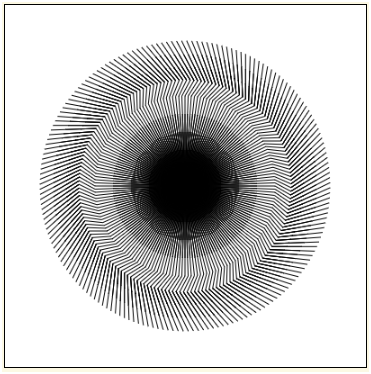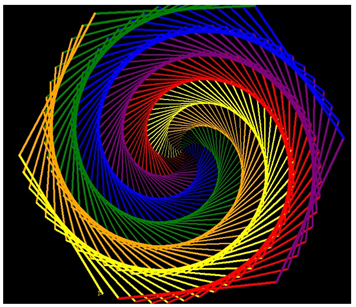Content

Python Turtle –

Turtle graphics is a special feature of python, with the help of which we can draw different types of patterns. From these libraries one can build more complex shapes like squares, triangles, circles and many composite patterns like Python Turtle Clock,

let's examine how it works-

Imagine that we have a turtle, who understands English. You can tell him to simple commands such as to go forward, backward, right, and left. In this way turtle lefts footprints at its end, which results in formation of a pattern. As you will see, you can make some pretty amazing drawings with this simple capability.

User-defined Classes –

Python provides a way to define new functions in our programs, it also provides a way to define new classes of objects. Later we will discuss that how to define functions, and much later, new classes of objects. For now, you just need to understand how to use them.

Instances –

The class which we are using in this section are Turtle and Screen, we create a new instance with a syntax that looks like a function call, Turtle(). The Python interpreter will know that Turtle is a class rather than a function,  So it will create a new instance of the class, and furthermore it will return it. In this section, we had to refer to the class as turtle.Turtle. Thus, in the programs, we wrote turtle.Turtle() to make a new turtle. We could also write turtle.Screen() to make a new window for our turtles to draw patterns.

Attributes –

Every instance have attributes , sometimes they are referred as variable instance. They just behave like variables in python. Assignment statements are used to assign values by using an= sign. Let’s take an example, if there are two instances in turtle class named – tex,pex. Then we can assign values to these attributes such as –

Eg

tex.price = 400

pex.price = 600

print(alex.price + tess.price)

The fillowing code will print an amount of 1000

Methods Used In Turtle –

Classes have associated methods, which are just a special kind of function. Consider the expression tess.forward(50) The interpreter first looks up tess and finds that it is an instance of the class Turtle.

After that it will look upto the attribute forward and will find that it is a method. Since there is a left parenthesis directly following, the interpreter invokes the method, passing 50 as a parameter.

Let’s Start Our First Program –

Example code -

Output –

Let’s discuss a couple of things about the program –

The first line will import the turtle library to the python. That module brings us two new types              that we can use: the Turtle type, and the Screen type.

The dot notation turtle.Turtle means “The Turtle type that is defined within the turtle module”.

In order to create a drawing/pattern, we will need a window or screen to draw. So we have                   created a screen named “wn”, and colored it with blue color.

After that pattern is drawn using forward, left methods.

At last, the color of pen is changed to white.

EXAMPLE CODE -

import turtle

wn = turtle.Screen()                    # Set up the window and its attributes

wn.bgcolor("lightgreen")

tess = turtle.Turtle()                  # create tess and set his pen width

tess.pensize(5)

alex = turtle.Turtle()                   # create alex

alex.color("hotpink")                 # set his color

tess.forward(80)                        # Let tess draw an equilateral triangle

tess.left(120)

tess.forward(80)

tess.left(120)

tess.forward(80)

tess.left(120)                              # complete the triangle

tess.right(180)                          # turn tess around

tess.forward(80)                      # move her away from the origin so we can see alex

alex.forward(50)                      # make alex draw a square

alex.left(90)

alex.forward(50)

alex.left(90)

alex.forward(50)

alex.left(90)

alex.forward(50)

alex.left(90)

OUTPUT -``

EXAMPLE -2

import turtle

wn = turtle.Screen()         #Set up the window and its attributes

wn.bgcolor("lightgreen")

tess = turtle.Turtle()       #create tess and set his pen width

for i in range(50):        #the loop will be executed for 50 times

tess.forward(100)

tess.right(144)

In this example tess(Turtle) is first moving 100px forward and then it takes right of angle 144°.

And then above steps are repeated 50 times, which results in the formation of a star.

Output-

Miscellaneous Examples –

Ninja Twist (Turtle Graphics) -

import turtle

ninja = turtle.Turtle()

ninja.speed(10)

for i in range(180):

ninja.forward(100)

ninja.right(30)

ninja.forward(20)

ninja.left(60)

ninja.forward(50)

ninja.right(30)

ninja.penup()

ninja.setposition(0, 0)

ninja.pendown()

ninja.right(2)

turtle.done()

Output -At the first move, the turtle is moved 100px then it turned 30° right after that again turtle is asked to move 20px.

Then it turned 60° right and further moved by distance 50px, in the last couple of lines the turtle is set to penup it’s tail & then it is sent to the initial position.

After reaching the initial position it is commanded to turn to be 2° by which new zig-zag line will be formed.

The above steps are performed repeatedly to form the desired pattern.

Example 2

# import turtle library

import turtle

wn = turtle.Screen()             # Set up the window and its attributes

wn.bgcolor("black")

colors = [ "red","purple","blue","green","orange","yellow"]

draw = turtle.Turtle()

for x in range(360):

draw.pencolor(colors[x % 6])

draw.width(x/100 + 1)

draw.forward(x)

draw.left(59)

turtle.done()

Output -Example  -

Python Turtle Clock –

In this example we have to crate a clock with a dial of color red and the outer circumference of the clock will be of blue color.

import turtle

screen=turtle.Screen()

clock=turtle.Turtle()

screen.setup(620,620)

screen.bgcolor('light green')

clr=['red','green','blue','yellow','purple']

clock.pensize(4)

clock.penup()

clock.pencolor('red')

m =0

for i in range(12):

m=m+1

clock.penup()

clock.forward(150)

clock.pendown()

clock.forward(25)

clock.penup()

clock.forward(20)

clock.write(str(m),align="center",font=("Arial", 12, "normal"))

if m==12:

m=0

clock.home()

clock.home()

clock.setpos(0,-250)

clock.pendown()

clock.pensize(10)

clock.pencolor('blue')

clock.circle(250)

clock.penup()

clock.setpos(150,-270)

clock.pendown()

clock.pencolor('olive')

clock.ht()

Output